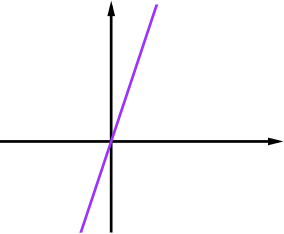### Combining Functions

Fluency exercise

The function $a(x)=x+1$ can be combined in a number of ways with each of the functions $b(x)=2x-1, \quad b(x)=x^2-5x+8, \quad b(x)=x^2-5x-6$ to create a set of new functions. What functions are created by adding, subtracting and multiplying? Can you sketch them in the cells of the grid below?
$a(x)+b(x)$ $a(x)-b(x)$ $a(x)\times b(x)$
$b(x)=$ $2x-1$$y=3x$
$b(x)=$ $x^2-5x+8$
$b(x)=$ $x^2-5x-6$